# How to use EDATE function in Excel with examples

In this tutorial, you will learn how to use EDATE formula in Excel and combine it with other functions for a variety of purposes beyond its basic use.

Microsoft Excel provides a variety of functions to calculate dates. When it comes to adding or subtracting months to/from a given date, EDATE is the function to use. Additionally, it can help you calculate expiration dates, anniversary dates, due dates, and a lot more.

## Excel EDATE function

The EDATE function in Excel returns a date on the same day of the month, n months before or after a given date.

The syntax has two arguments:

EDATE(start_date, months)

Where:

• Start_date (required) - the start date in the form of a valid Excel date. It can be supplied as a text string in the format that Excel interprets as a date (e.g. "10-Jan-2025"), as a reference to a cell containing a date, or as a result of another function such as DATE(2025,1,10).
• Months (required) - the number of months to add to or subtract from the start date. A positive value returns a future date, a negative value - a past date.

And here are a few examples of using the EDATE function in Excel: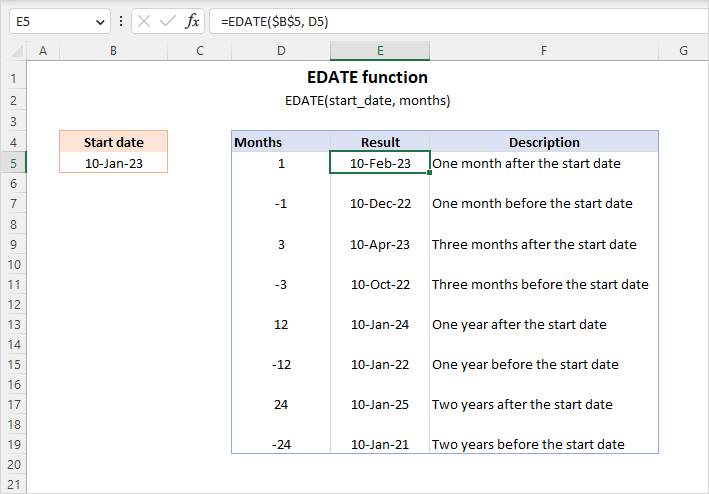Note. By default, the EDATE function returns a serial number representing a date internally in Excel. To display the result as a date, apply a Date format of your choosing.

## How to use EDATE formula in Excel

To make a basic EDATE formula in Excel, follow these steps:

1. For the start_date­ argument, supply a valid Excel date in one of these forms:
• a string enclosed in quotation marks, e.g. "1/30/2025" or "30-Jan-2025"
• a DATE formula, e.g. DATE(2025, 1, 30)
• a reference to the cell containing the start date
2. For the months argument, provide an integer specifying how many months to add to or subtract from the start date. To shift the date forward, use a positive number - the output will be a future date. To move the date backwards, supply a negative number - the result will be a past date.
3. Apply the desired DATE format to the formula cell.

For example, to return a date 5 months after 30 January 2025, use one of these formulas:

`=EDATE("1/30/2025", 5)`

`=EDATE("30-Jan-2025", 5)`

`=EDATE(DATE(2025, 1, 30), 5)`

To get a date 5 months before 30 January 2025, the formulas go as follows:

`=EDATE("1/30/2025", -5)`

`=EDATE("30-Jan-2025", -5)`

`=EDATE(DATE(2025, 1, 30), -5)`

With the start date in a predefined cell, say C4, the formulas are:

`=EDATE(C4, 5)`

`=EDATE(C4, -5)`

The result is a date on the same day of the month, a specified number of months before or after the initial date.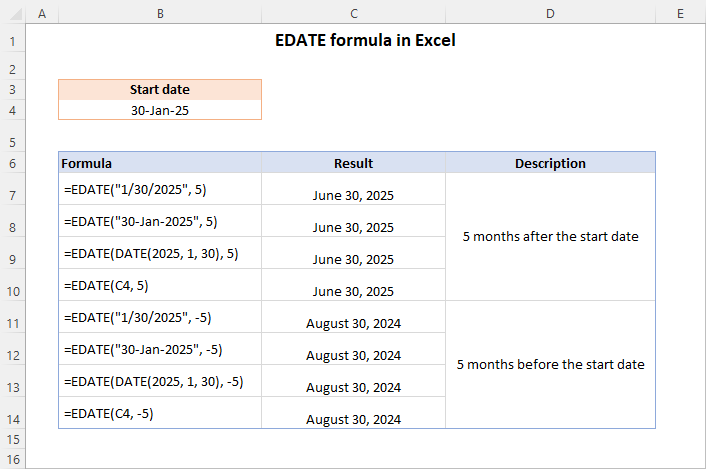Tips and notes

The EDATE function falls under the category of DATE and TIME functions. Dates in Excel may have different formats, but they are actually stored as numbers. Only valid Excel dates can be used in calculations.

• If start_date is not a valid date, an EDATE formula returns a #VALUE! error.
• If the start_date value has a fractional part representing time, it will be truncated. To force EDATE to keep time, use this formula.
• If the months argument is not an integer, its decimal part will be removed.
• The EDATE function returns a serial number corresponding to the date (General format). For the result to display correctly, it must be formatted as a date.

With the Excel EDATE basics in mind, let's drill into a few practical examples that highlight particular aspects and show how to accomplish some common tasks.

## How to get a date n months from today

To return a date n months before or after today's date, use EDATE in combination with the TODAY function:

EDATE(TODAY(), months)

For example, to find a date that is 3 months from today, you can use this formula:

`=EDATE(TODAY(), 3)`

To get a date exactly 3 months before today, the formula is:

`=EDATE(TODAY(), -3)`

More formula examples are shown in the screenshot below: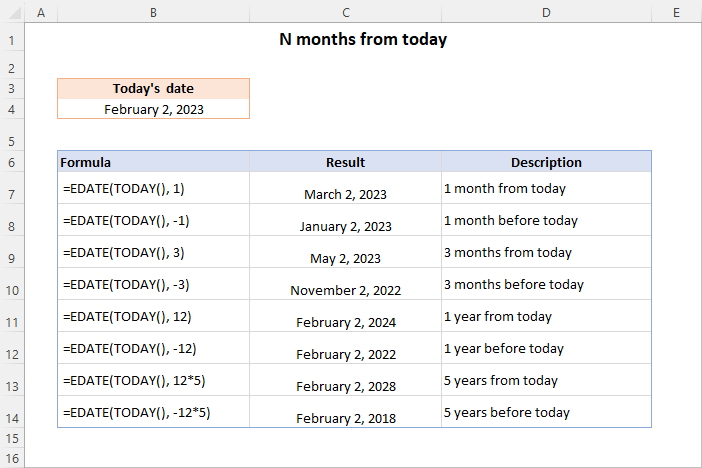## How to calculate n years from date

To calculate a date shifted a certain number of years in future or past, you can multiply the number of years by 12:

EDATE(start_date, years*12)

For instance, to move a date 10 years forward from the date in A3, you can use one of these formulas:

`=EDATE(A3, 120)`

`=EDATE(A3, 10*12)`

To move a date 10 years backward, the formulas are:

`=EDATE(A3, -120)`

`=EDATE(A3, -10*12)`

The first form is more contact while the second variation is more convenient to use when you have a number of years in another cell. For example:

`=EDATE(A3, B3*12)`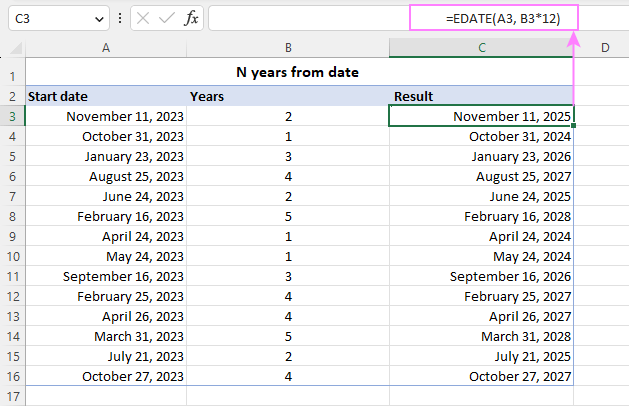## EDATE formula to get last day of month

The EDATE function correctly returns the last day of month if the start day is 31.

For example, the formula below returns 30-Apr-2023, which is a date 3 months after 31-Jan-2023:

`=EDATE("31-Jan-2023", 3)`

It also maintains the last day of February in leap years. For instance, the result of this formula is 29-Feb-2024:

`=EDATE("31-Dec-2023", 2)`

However, if the start day value is less than 31, EDATE will return a date exactly on the same day of the month in the past or future, as per its main purpose. In this case, you can compute the last day of month by using the EOMONTH function. For example:

`=EOMONTH("26-May-2023", 3)`

The screenshot below shows the results of both formulas - the wrong ones are in the red font color: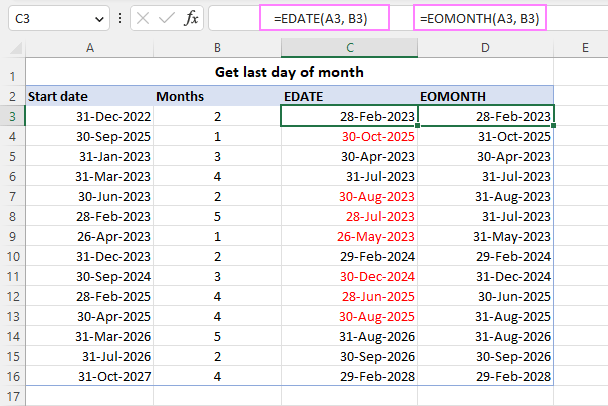## EDATE formula to calculate expiry dates

Knowing the start date and duration in months, calculating an expiry, due, anniversary or retirement date is as easy as pie.

All you have to do is to supply the start date in the first argument of EDATE and the number of months in the second argument. For example, with the start date in A3 and months in B3, the formula is:

`=EOMONTH(A3, B3)`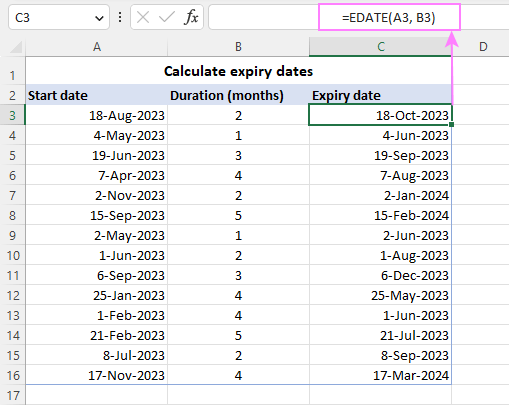## EDATE formula inside of Excel IF statement

In situation when you need to add or subtract a different number of months to/from a date based on one or more conditions, you can nest an EDATE formula inside of an IF statement.

Let's say you want to calculate an expiry date based on the subscription type - monthly or annual. To have it done, you construct the following nested IF formula:

`=IF(B3="monthly", EDATE(A3, 1), IF(B3="annual", EDATE(A3, 12), "N/A"))`

If B3 contains the word "monthly", the first EDATE function adds one month to the start date in A3. If B3 has "annual", another EDATE function adds 12 months to the start date. If B3 contains anything else or is blank, the formula returns "N/A".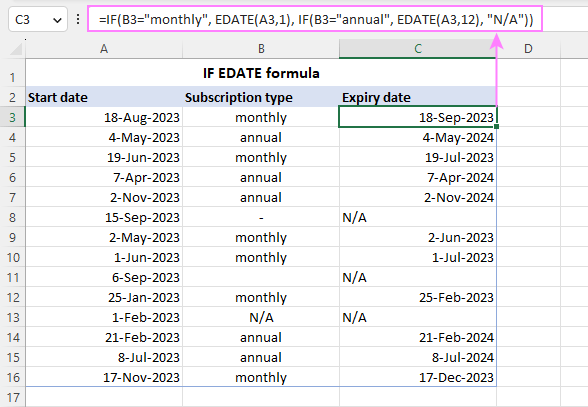## EDATE to keep time value

When the start date value also includes time, the EDATE function drops the time part. To preserve time, extract it from the datetime value with the help of the MOD function, and then add to the EDATE's result:

EDATE(start_date, months) + MOD(start_date, 1)

For instance, to add a certain number of months (B3) to a datetime value in A3 preserving the time, use this formula:

`=EDATE(A3, B3) + MOD(A3, 1)`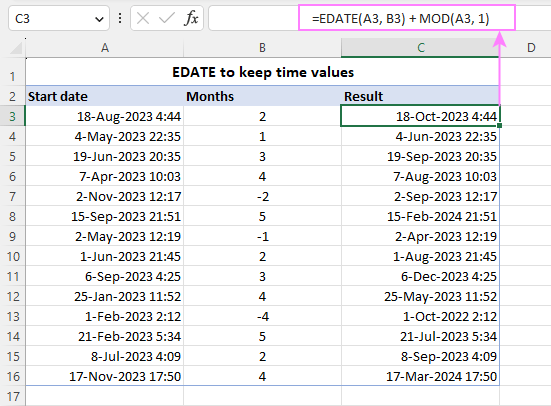That's how to use the EDATE function in Excel to add or subtract months to/from a given date. Thank you for reading and see you on our blog next week!

Using EDATE formula in Excel - examples (.xlsx file)

## You may also be interested in

1. How to extract? the Day (Number, not text, etc.), the Year, and the Month (text, not number) of. date cell.

2. Hi, I am trying to apply the EDATE formula with the below mentioned formula
=IF(F2="Yearly",EDATE(G2,1*12),IF(F2="Quarterly",EDATE(G2,3),IF(F2="Half Yearly",EDATE(G2,6),"-")))

the problem i am facing is even though the f column shows the text as Yearly or half yearly, I only end up getting "-" as my output.

• Hi! Unfortunately, I could not reproduce your problem. Pay attention to cell F2 - extra spaces or other unprintable characters.

3. Hello,

I'm attempting to use the following example in my sheet: =IF(B3="monthly", EDATE(A3, 1), IF(B3="annual", EDATE(A3, 12), "N/A")). I'm good with the results IF I'm adding or subtracting whole months. However, is there a way with EDATE to add or subtract fractions of months.

For example, I'm trying to calculate when a renewal date should happen. My options are 30 days from original start date, 45 days from original start date or 60 days from original start date. The formula I've built is working great for both the 30 and 60 day examples (my hunch is because they are whole months - a factor of 1 or 2). However, when I try to build it out for the 45 day example using a factor of 1.5, it's defaulting to 1.

Is there a way to add/subtract half-months using EDATE?

I appreciate the information on this blog - it's been extremely helpful.

Paul

4. Thanks for the practice file.
Without practice it becomes difficult to practice.